Tags: ohbm2018

# Solving the EEG and MEG forward problem using the finite element method

## Introduction

The aim of this tutorial is to solve the EEG and MEG forward problems using the Finite Element Method (FEM).

## Background

The EEG/MEG signals measured on or around the scalp do not directly reflect the activated neurons in the brain. To reconstruct the actual activity in the brain, source reconstruction techniques are used. You can read more about the different methods in the review papers that are listed here.

The activity in the brain is estimated from the EEG or MEG signals using

1. the EEG/MEG activity itself that is measured on or around the scalp
2. the spatial arrangement of the electrodes/gradiometers relative to the brain (sensor positions),
4. the location of the source (source model)

Using this information, source estimation comprises two major steps: (1) Estimation of the EEG potential or MEG field distribution for a known source is referred to as forward modeling. (2) Estimation of the unknown sources corresponding to the measured EEG or MEG is referred to as inverse modeling.

The forward solution can be computed when the head model, the sensor positions and a model for the source are given. For distributed source models and for scanning approaches such as beamforming, the source model consists of a discrete description of the the brain volume or of the cortical sheet in many voxels or vertices. When the forward solution is computed, the lead field matrix (with dimensions Nchan * Nsources) is calculated for each point, taking into account the head model and the sensor positions.

A prerequisite of forward modeling is that the geometrical description of the sensor positions, head model and source model are expressed in the same coordinate system (e.g., CTF, MNI, Talairach) and with the same units (mm, cm, or m). There are different conventions for coordinate systems. The precise coordinate system is not relevant, as long as all data is consistent. Here you can read how the different head and MRI coordinate systems are defined. For most MEG systems, the gradiometers are by default defined relative to head localizer coils or anatomical landmarks, therefore when the anatomical MRI are aligned to the same landmarks, the position of the MEG sensors directly matches the MRI. As EEG data is typically not explicitly aligned relatively to the head, therefore, the EEG electrodes usually have to be explicitly realigned prior to source reconstruction (see also this faq and this example).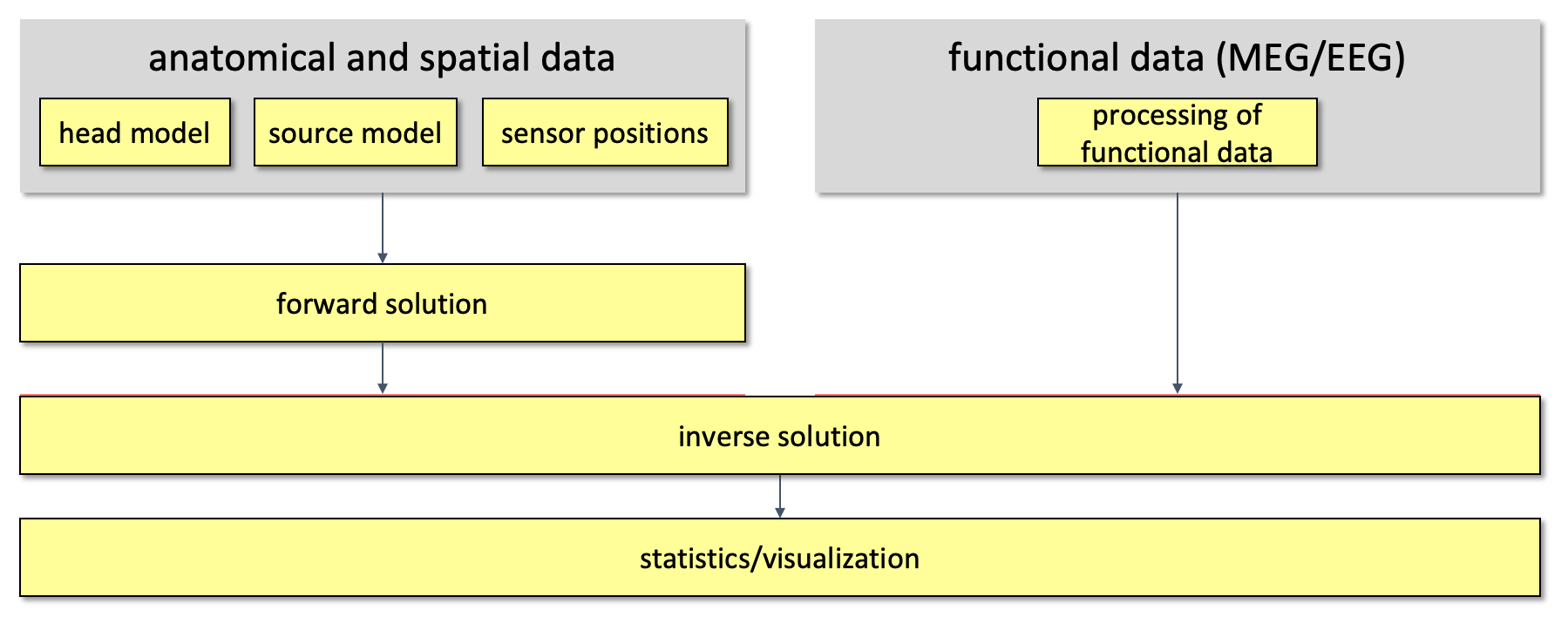Figure 1. Overall outline of the pipeline used for source reconstruction

## Procedure

As already mentioned, the goal of this session is to solve the EEG and MEG forward problem, more precisely we want to compute EEG and MEG leadfields so that the inverse problem can be solved in the next session (inverse problem).

To compute the leadfields, there are five main steps that have to be followed.

1. create the mesh: in this step the MRI is loaded and processed, the segmentation is performed and finally the mesh is generated;
2. create the headmodel: in this step the geometrical (mesh) and electrical (tissue conductivities) features are merged together;
3. create the sourcemodel: in this step a grid of source positions in the gray matter is created;
4. handle the sensors: loading the electrodes and the gradiometers and aligning the electrodes to the scalp surface if needed;
5. compute the leadfield, i.e., solve the forward problem: this steps consists of two procedures. First, the so-called transfer matrix is computed; second, the leadfield matrix is estimated.

The first step is the same for solving both the EEG and MEG forward problem, the other four have to be executed separately for EEG and MEG. See Figure1.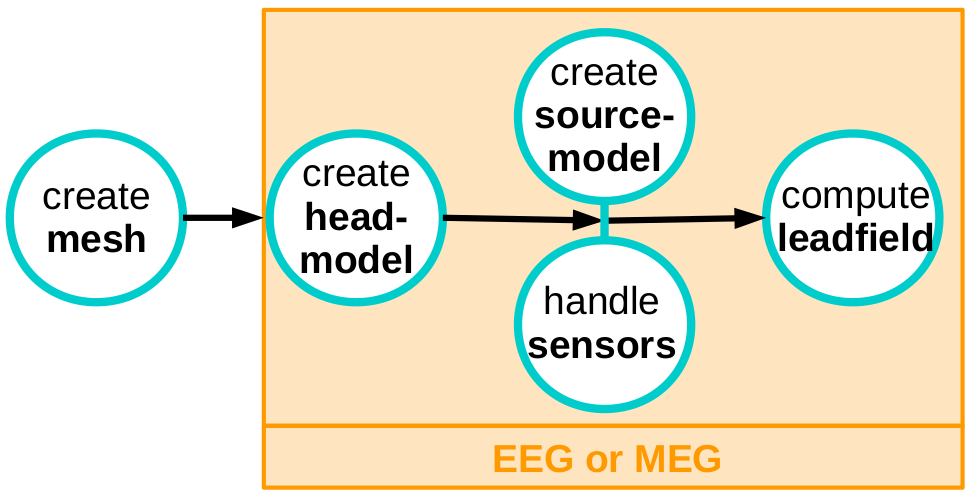Figure: pipeline for forward computation using FEM, in the orange box there are the steps which differ between EEG and MEG

In particular, the EEG forward solution is computed via the method so-called simbio which relies on the code that you can find here, while the MEG forward solution calls the duneuro method, which makes use of the code developed in the University of Münster, visit this for further details.

The integration of SimBio with FieldTrip is described in the reference below. Please cite this reference if you use the FieldTrip-SimBio pipeline in your research.

Vorwerk, J., Oostenveld, R., Piastra, M.C., Magyari, L., & Wolters, C. H. The FieldTrip‐SimBio pipeline for EEG forward solutions. BioMed Eng OnLine (2018) 17:37. DOI: 10.1186/s12938-018-0463-y.

## 1. Create the mesh

This procedure consists in six steps. The input is a T1 weighted MRI and the output is a volumetric mesh with five compartments, i.e., scalp, skull, cerebrospinal fluid (CSF), gray and white matter.

``````mri_orig = ft_read_mri('subject01.nii');
``````

Visualize the MRI

``````cfg = [];
ft_sourceplot(cfg,mri_orig);
``````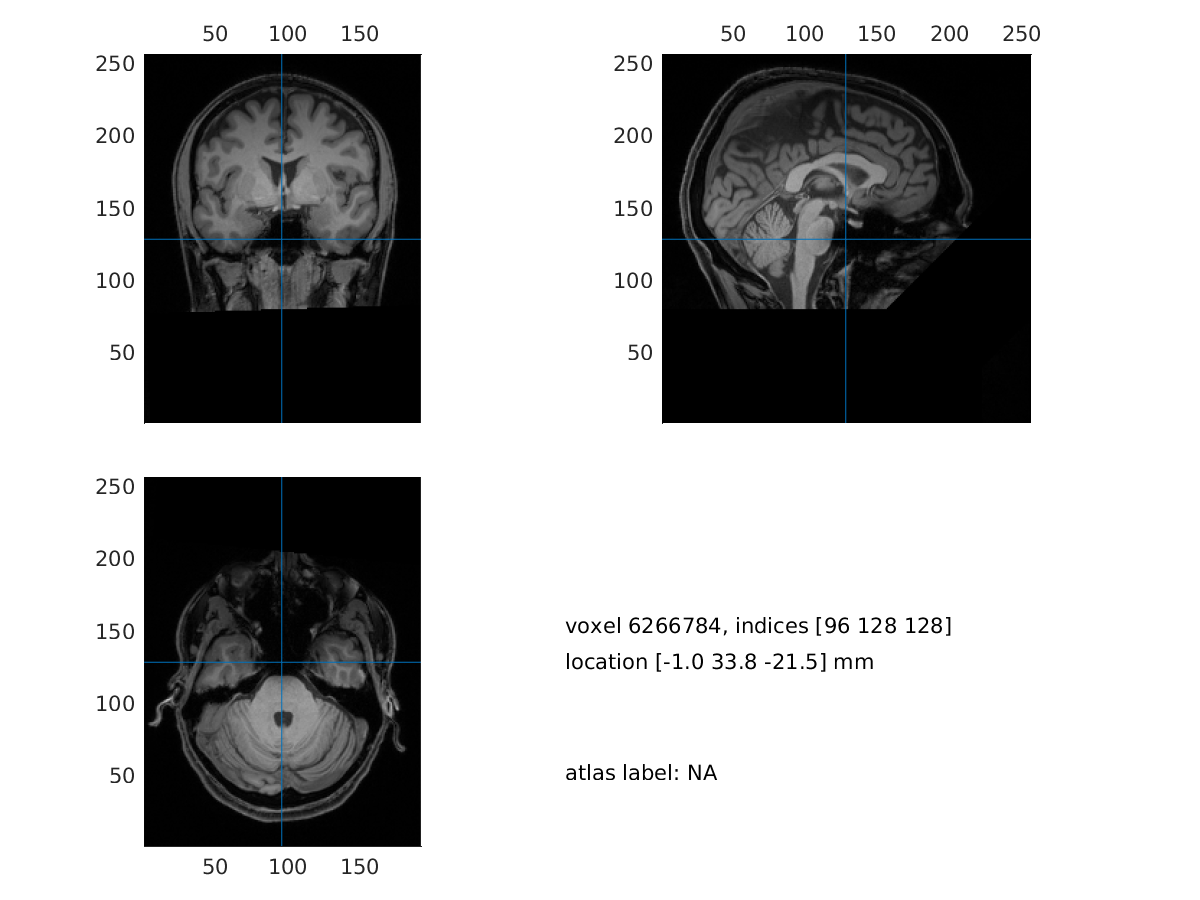Figure: visualization of the MRI

### b. realign the MRI

In this step we will interactively align the MRI to the CTF space. We will be asked to identify the three CTF landmarks (nasion, NAS; right pre-auricular point, RPA; left pre-auricular point, LPA) in the MRI.

``````cfg           = [];
cfg.method    = 'interactive';
cfg.coordsys  = 'ctf';
mri_realigned = ft_volumerealign(cfg, mri_orig);
``````

We can visualize the realigned MRI

``````cfg = [];
ft_sourceplot(cfg, mri_realigned);
``````

### c. reslice the MRI

``````cfg          = [];
mri_resliced = ft_volumereslice(cfg, mri_realigned);
``````

We can visualize the resliced MRI

``````cfg = [];
ft_sourceplot(cfg, mri_resliced);
``````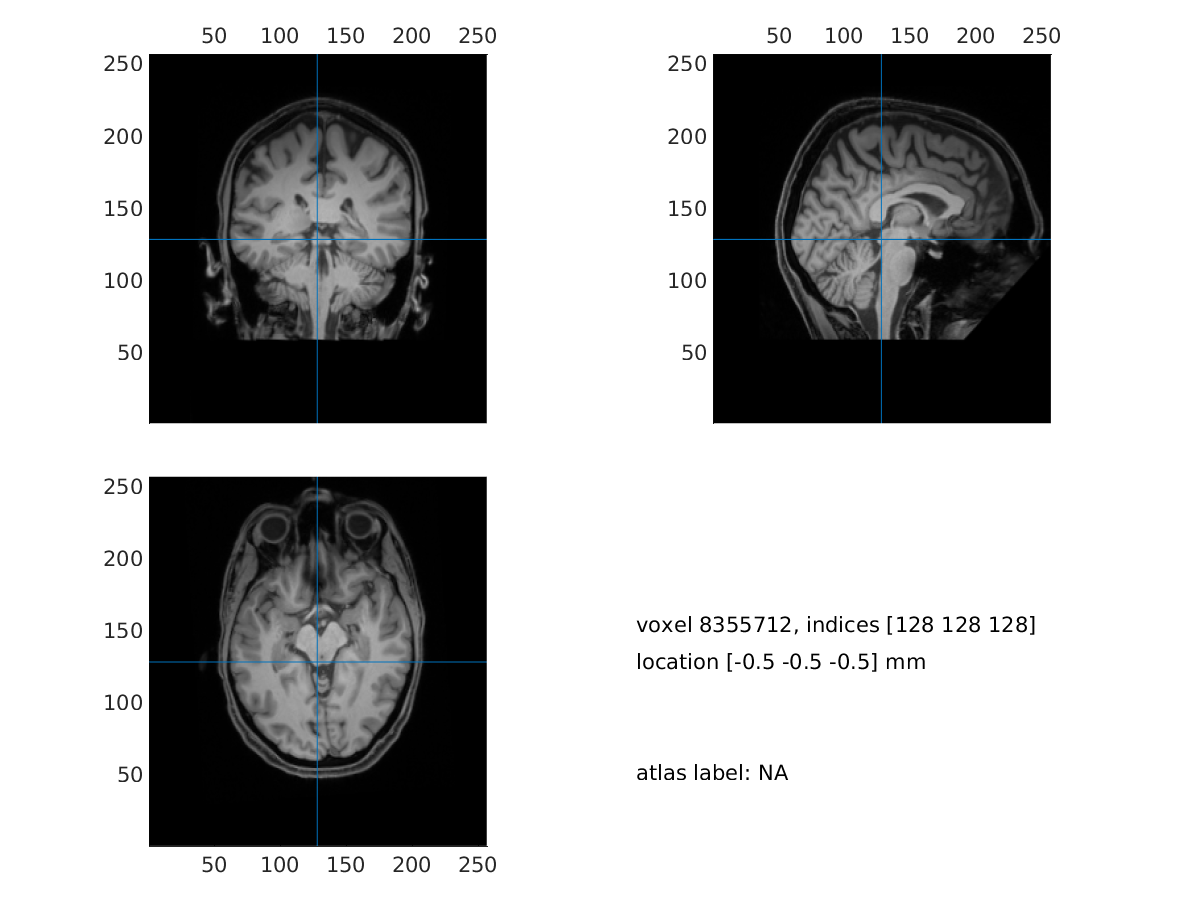Figure: visualization of the replaced MRI

### d. create surface meshes

For visualization purposes, we produce surface meshes for three compartments: scalp, skull and brain. In order to do that, we first have to segment the MRI into the three compartments.

``````cfg                         = [];
cfg.output                  = {'scalp', 'skull', 'brain'};
mri_segmented_3_compartment = ft_volumesegment(cfg, mri_resliced);
``````

Visualize the segmentation

``````seg_i = ft_datatype_segmentation(mri_segmented_3_compartment, 'segmentationstyle', 'indexed');

cfg              = [];
cfg.funparameter = 'seg';
cfg.funcolormap  = gray(4); % distinct color per tissue
cfg.location     = 'center';
cfg.atlas        = seg_i;
ft_sourceplot(cfg, seg_i);
``````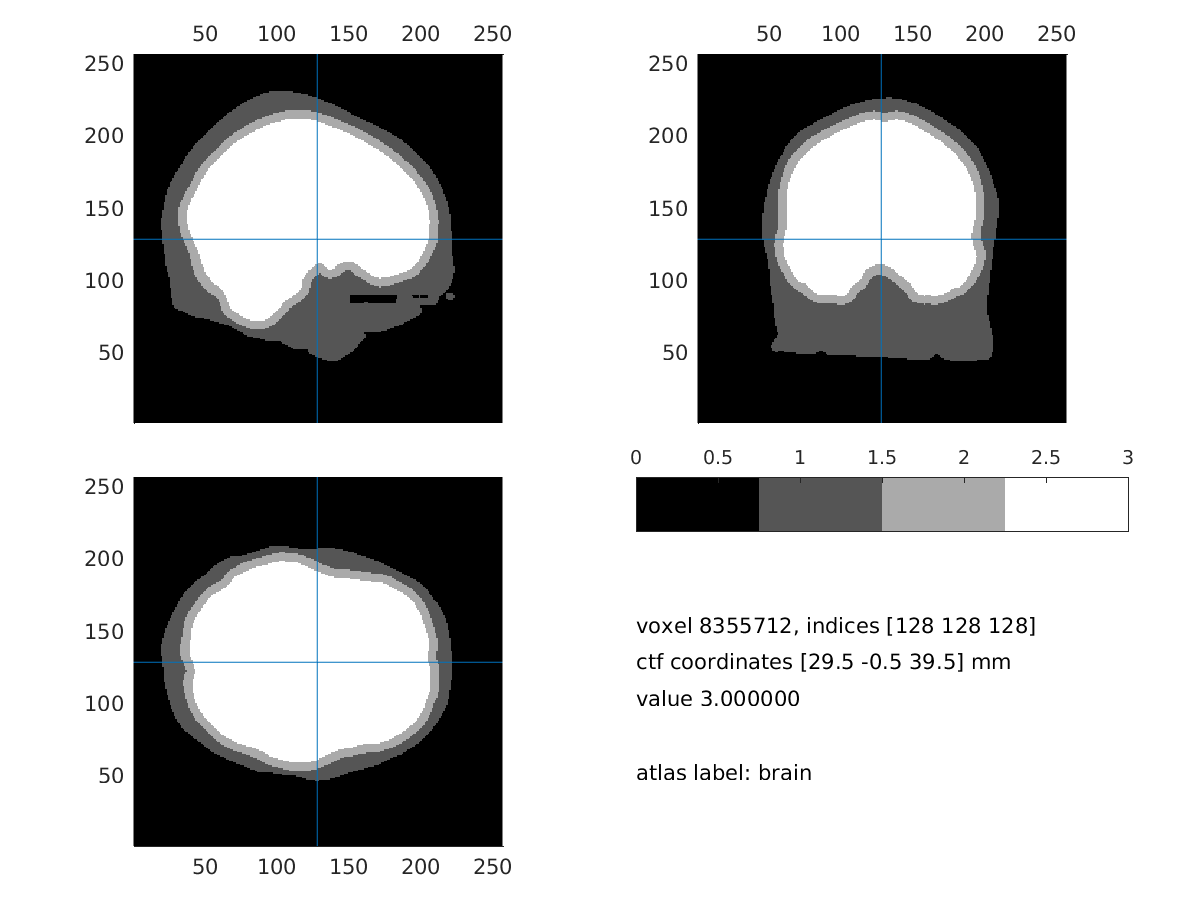Figure: three compartment segmentation output

Once the segmentation is completed, the three surface meshes can be computed.

``````cfg  =[];
cfg.tissue      = {'scalp', 'skull', 'brain'};
cfg.numvertices = [3000 2000 1000];
mesh_surf       = ft_prepare_mesh(cfg,mri_segmented_3_compartment);
``````

We can save the mesh obtained

``````save mesh_surf mesh_surf
``````

### e. segment the MRI

``````cfg                         = [];
cfg.output                  = {'scalp', 'skull', 'csf', 'gray', 'white'};
cfg.brainsmooth             = 2;
cfg.skullsmooth             = 2;
cfg.scalpsmooth             = 2;
mri_segmented_5_compartment = ft_volumesegment(cfg, mri_resliced);
``````

Visualize the segmentation result

``````seg_i = ft_datatype_segmentation(mri_segmented_5_compartment, 'segmentationstyle', 'indexed');

seg_i = ft_datatype_segmentation(mri_segmented_5_compartment, 'segmentationstyle', 'indexed');

cfg              = [];
cfg.funparameter = 'seg';
cfg.funcolormap  = gray(6); % distinct color per tissue (air is included)
cfg.location     = 'center';
cfg.atlas        = seg_i;    % the segmentation can also be used as atlas
ft_sourceplot(cfg, seg_i);
``````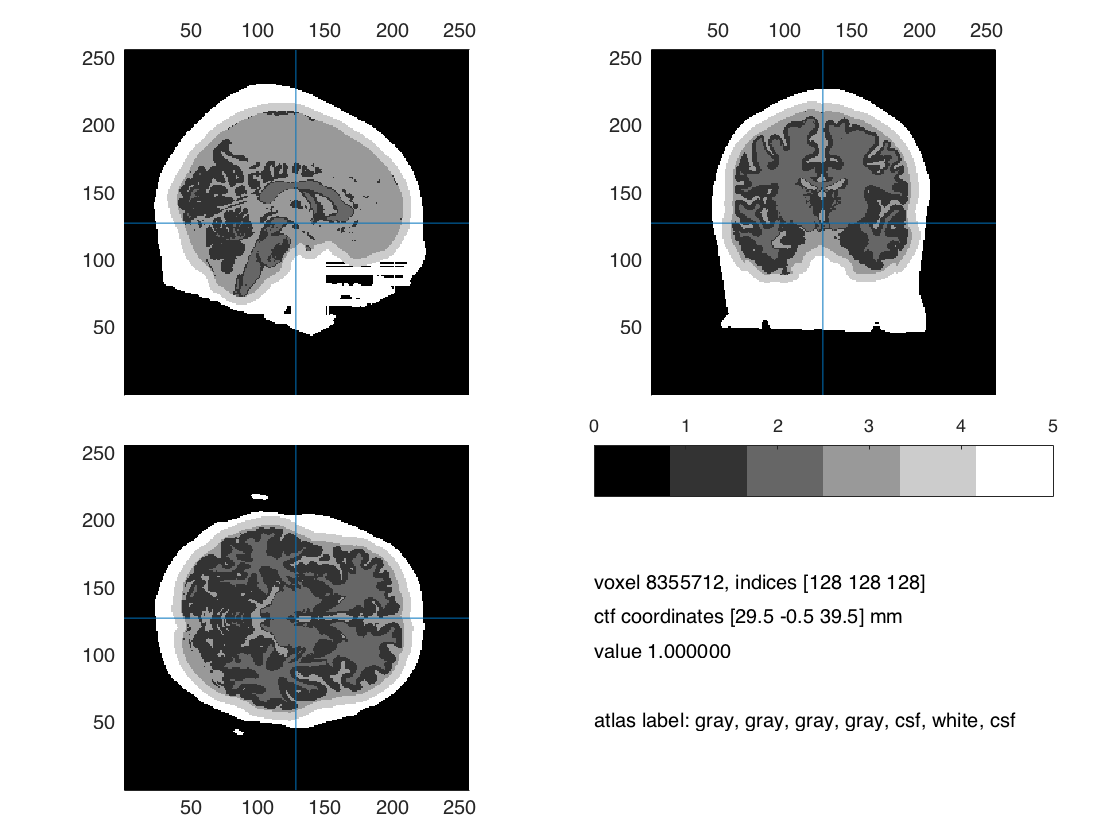Figure: 5 compartment segmentation output.

### f. create the mesh

``````cfg            = [];
cfg.shift      = 0.3;
cfg.method     = 'hexahedral';
cfg.resolution = 2; % this is in mm
cfg.tissue     = {'scalp', 'skull', 'csf', 'gray', 'white'};
mesh_fem       = ft_prepare_mesh(cfg,mri_segmented_5_compartment);
``````

For this tutorial we downsample the mesh to 2mm resolution, in order to reduce the computation time of the following steps.

## EEG and MEG forward solution computation

Once the volumetric mesh has been created, the forward solution can be computed. In the following, steps 2-5 are described for EEG and MEG separately.

Currently, the pipeline for computing the MEG forward problem solution has been tested on Ubuntu systems, where MATLAB should be started with the following command:

``````BLAS_VERSION=/usr/lib/libblas.so
./matlab
``````

``````cfg               = [];
cfg.method        = 'simbio';
cfg.conductivity  = [0.43 0.01 1.79 0.33 14];
cfg.tissuelabel   = {'scalp', 'skull', 'csf', 'gray', 'white'};
``````

Visualize the headmodel and the electrodes (it might take time and memory)

``````% scalp: 1, skull: 2, csf: 3, gray: 4, wm: 5
ts = 1;
figure
mesh2 =[];

mesh_ed = mesh2edge(mesh2);
patch('Faces',mesh_ed.poly,...
'Vertices',mesh_ed.pos,...
'FaceAlpha',.5,...
'LineStyle', 'none',...
'FaceColor',[1 1 1],...
'FaceLighting', 'gouraud');

xlabel('coronal');
ylabel('sagital');
zlabel('axial')
camlight;
axis on;

ft_plot_sens(elec, 'style', '*g');
``````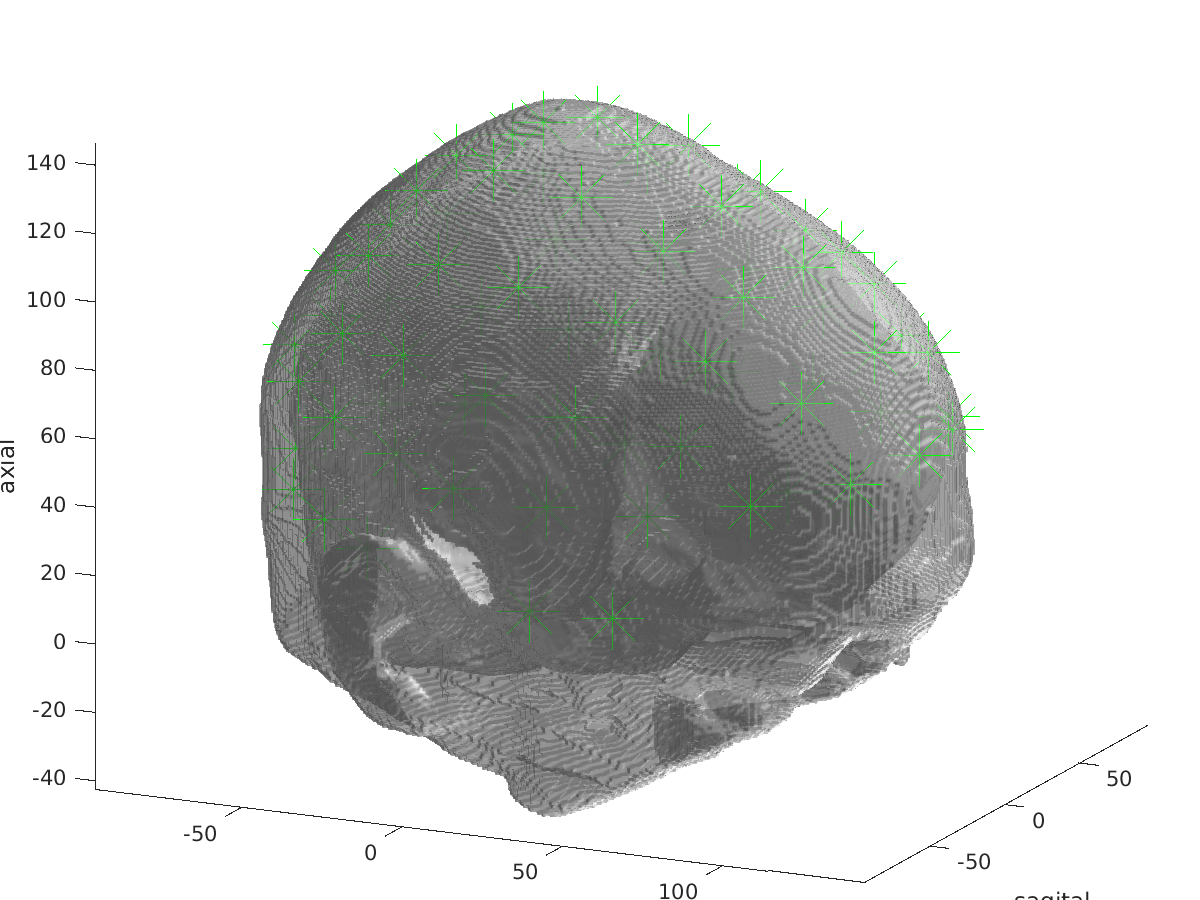Figure: visualization of headmodel_fem_eeg and electrodes

## 3(EEG). Create the sourcemodel

In this phase, source locations are selected within the gray matter compartment. During this tutorial we recommend to create a rather coarse grid (cfg.resolution = 5;), in order to be able to compute the forward solution in the time available in this course.

``````cfg                 = [];
cfg.resolution = 5; %in mm
cfg.inwardshift     = 1; %shifts dipoles away from surfaces
``````

We can visualize the sources and the scalp surface mes

``````figure, ft_plot_mesh(sourcemodel.pos(sourcemodel.inside,:))
hold on, ft_plot_mesh(mesh_surf(1), 'surfaceonly', 'yes', 'vertexcolor', 'none', 'facecolor',...
'skin', 'facealpha',0.5, 'edgealpha',0.1)
``````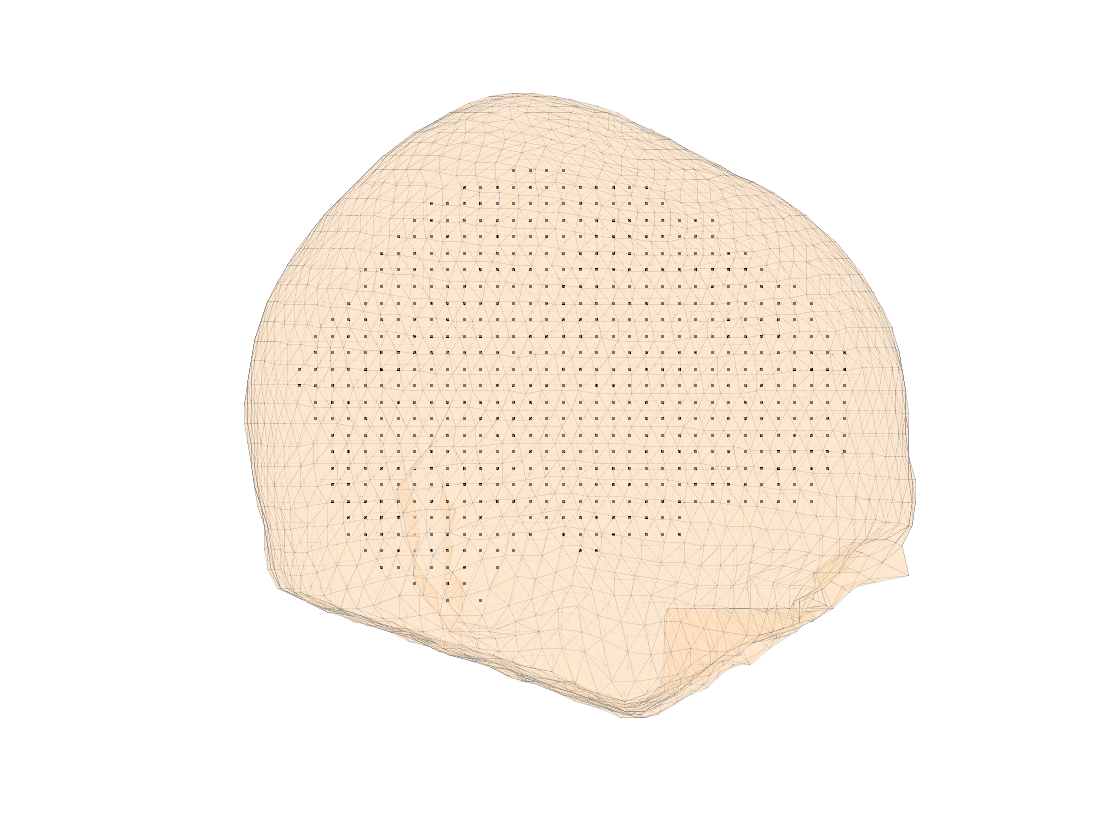Figure: visualization of the source model

## 4(EEG). Handle the sensors

In case the electrodes are not aligned to the MRI (i.e., CTF space), we can use the interactive function as follows

``````cfg           = [];
cfg.method    = 'interactive';
cfg.elec      = elec;
elec  = ft_electroderealign(cfg);
``````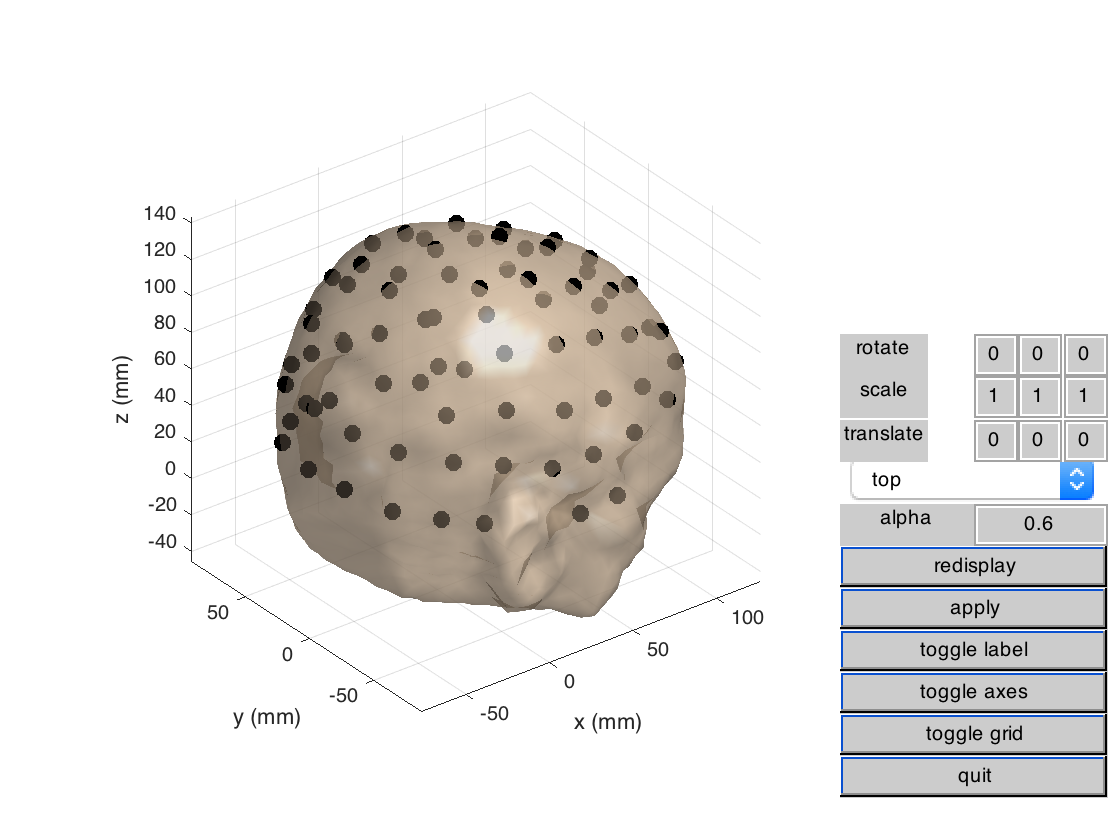Figure: visualization of headmodel_fem_eeg and electrodes

Please DO NOT run ft_prepare_vol_sens in this tutorial session! It will take too much time and memory. Load “headmodel_fem_eeg_tr”.

``````%% compute the transfer matrix

cfg               = [];
cfg.grid          = sourcemodel;
cfg.elec          = elec;
cfg.reducerank    = 3;
``````

``````cfg               = [];
cfg.method        = 'duneuro';
cfg.conductivity  = [0.43 0.01 1.79 0.33 0.14]; % check that the order is the same as the one i the mesh_fem
``````

## 3(MEG). Create the sourcemodel

If the sourcemodel was already created at the step 3(EEG), it can be simply loaded for this step.

``````cfg                 = [];
cfg.resolution = 5; %in mm
cfg.inwardshift     = 1; %shifts dipoles away from surfaces
``````

We can visualize the sources and the scalp surface mes

``````figure, ft_plot_mesh(sourcemodel.pos(sourcemodel.inside,:))
hold on, ft_plot_mesh(mesh_surf(1), 'surfaceonly', 'yes', 'vertexcolor', 'none', 'facecolor',...
'skin', 'facealpha',0.5, 'edgealpha',0.1)
``````

## 4(MEG). Handle the sensors

As already mentioned, the MRI was realigned to the CTF space, therefore there is no need to realign the sensors. We load them from the data.

``````hdr     = ft_read_header('subject01.ds', 'headerformat', 'ctf_ds');
``````

We can visualize both EEG and MEG sensors, together with the scalp surface mesh

``````figure
hold on
ft_plot_mesh(mesh_fem, 'surfaceonly', 'yes', 'vertexcolor', 'none', 'edgecolor', 'none', 'facecolor',[0.5 0.5 0.5], 'facealpha',0.1, 'edgealpha', 0.1);
camlight
axis on
ft_plot_sens(elec);
``````

Please DO NOT run `ft_prepare_vol_sens` in this tutorial session! It will take too much time and memory. Load the file `headmodel_fem_eeg_tr.mat` from disk.

``````%% compute the transfer matrix

cfg                = [];
cfg.grid           = sourcemodel;
cfg.reducerank     = 2;
``````

## Exercises

#### Exercise 1

Realign the electrodes in the file `elec_shifted.mat` to the headmodel you created.

#### Exercise 2

Compute a finer sourcemodel, e.g., 2 mm resolution and compute the respective EEG and MEG forward solutions.

#### Exercise 3

Compute the EEG and MEG forward solution using the Boundary Element Method (BEM), e.g., following this tutorial.